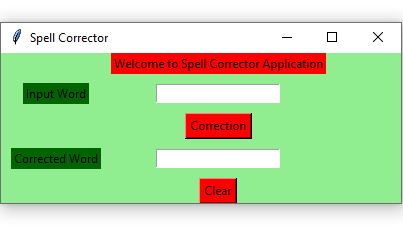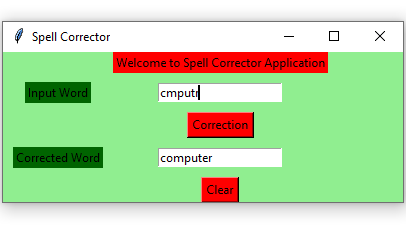# Python – Spell Corrector GUI using Tkinter

Prerequisites : Introduction to tkinter | spell checking

Python offers multiple options for developing a GUI (Graphical User Interface). Out of all the GUI methods, tkinter is the most commonly used method. Python with tkinter outputs the fastest and easiest way to create GUI applications. In this article, we will learn how to create a GUI Spell Corrector application using Tkinter, with a step-by-step guide.

To create a tkinter :

• Importing the module – tkinter
• Create the main window (container)
• Add any number of widgets to the main window.
• Apply the event Trigger on the widgets.

Let’s create a GUI based Spell Corrector application that can correct the word given by the user.

Below is the implementation :

 `# import all functions / classes from the tkinter   ` `from` `tkinter ``import` `*` `from` `textblob ``import` `TextBlob ` ` `  `# Function to clear both the text entry boxes ` `def` `clearAll() : ` `     `  `    ``# whole content of text entry area  is deleted  ` `    ``word1_field.delete(``0``, END) ` `    ``word2_field.delete(``0``, END) ` ` `  `# Function to get a corrected word ` `def` `correction() : ` ` `  `    ``# get a content from entry box ` `    ``input_word ``=` `word1_field.get() ` ` `  `    ``# create a TextBlob object ` `    ``blob_obj ``=` `TextBlob(input_word) ` ` `  `    ``# get a corrected word ` `    ``corrected_word ``=` `str``(blob_obj.correct()) ` ` `  `    ``# insert method inserting the   ` `    ``# value in the text entry box. ` `    ``word2_field.insert(``10``, corrected_word) ` ` `  ` `  `# Driver code  ` `if` `__name__ ``=``=` `"__main__"` `:  ` ` `  `    ``# Create a GUI window  ` `    ``root ``=` `Tk() ` ` `  `    ``# Set the background colour of GUI window   ` `    ``root.configure(background ``=` `'light green'``)   ` `     `  `    ``# Set the configuration of GUI window (WidthxHeight)  ` `    ``root.geometry(``"400x150"``)   ` ` `  `    ``# set the name of tkinter GUI window   ` `    ``root.title(``"Spell Corrector"``) ` `     `  `    ``# Create Welcome to Spell Corrector Application: label   ` `    ``headlabel ``=` `Label(root, text ``=` `'Welcome to Spell Corrector Application'``,   ` `                      ``fg ``=` `'black'``, bg ``=` `"red"``)   ` `   `  `    ``# Create a "Input Word": label   ` `    ``label1 ``=` `Label(root, text ``=` `"Input Word"``,  ` `                 ``fg ``=` `'black'``, bg ``=` `'dark green'``)  ` `       `  `    ``# Create a "Corrected Word": label   ` `    ``label2 ``=` `Label(root, text ``=` `"Corrected Word"``,   ` `                   ``fg ``=` `'black'``, bg ``=` `'dark green'``)   ` `     `  `   `  `    ``# grid method is used for placing   ` `    ``# the widgets at respective positions   ` `    ``# in table like structure . ` `    ``# padx keyword argument used to set paading along x-axis . ` `    ``headlabel.grid(row ``=` `0``, column ``=` `1``)   ` `    ``label1.grid(row ``=` `1``, column ``=` `0``)   ` `    ``label2.grid(row ``=` `3``, column ``=` `0``, padx ``=` `10``)  ` ` `  `       `  `    ``# Create a text entry box   ` `    ``# for filling or typing the information.   ` `    ``word1_field ``=` `Entry()  ` `    ``word2_field ``=` `Entry() ` `        `  `    ``# padx keyword argument used to set paading along x-axis . ` `    ``# pady keyword argument used to set paading along y-axis .   ` `    ``word1_field.grid(row ``=` `1``, column ``=` `1``, padx ``=` `10``, pady ``=` `10``)   ` `    ``word2_field.grid(row ``=` `3``, column ``=` `1``, padx ``=` `10``, pady ``=` `10``)  ` ` `  `       `  `    ``# Create a Correction Button and attached   ` `    ``# with correction function   ` `    ``button1 ``=` `Button(root, text ``=` `"Correction"``, bg ``=` `"red"``, fg ``=` `"black"``,  ` `                                ``command ``=` `correction)  ` `       `  `    ``button1.grid(row ``=` `2``, column ``=` `1``)  ` `   `  `    ``# Create a Clear Button and attached   ` `    ``# with clearAll function   ` `    ``button2 ``=` `Button(root, text ``=` `"Clear"``, bg ``=` `"red"``,   ` `                     ``fg ``=` `"black"``, command ``=` `clearAll) ` `     `  `    ``button2.grid(row ``=` `4``, column ``=` `1``)  ` `     `  `    ``# Start the GUI   ` `    ``root.mainloop()   `

OUTPUT :My Personal Notes arrow_drop_upCheck out this Author's contributed articles.

If you like GeeksforGeeks and would like to contribute, you can also write an article using contribute.geeksforgeeks.org or mail your article to contribute@geeksforgeeks.org. See your article appearing on the GeeksforGeeks main page and help other Geeks.

Please Improve this article if you find anything incorrect by clicking on the "Improve Article" button below.

Article Tags :

Be the First to upvote.

Please write to us at contribute@geeksforgeeks.org to report any issue with the above content.